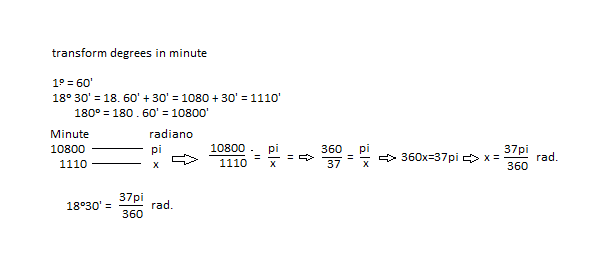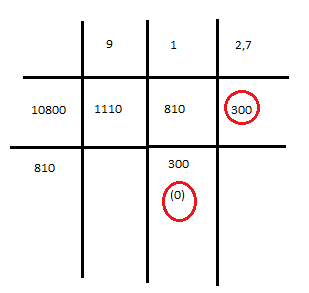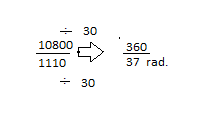# Circles -- transform degrees in minute

• B
A minha pergunta é na divisão 10800/1110
My question is in the division 10800/1110 ???Last edited by a moderator:

## Answers and Replies

jedishrfu
Mentor
They started with the notion that 180 degrees = ##\pi## radians

and then the equivalent notion that 10800 minutes = ##\pi## radians

and then they construct a ratio 180 deg / (18 deg 30 min) = (10800 min) / (1100 min) = 360 / 37 = ##\pi## / x radians

and then solve for x.

They started with the notion that 180 degrees = ##\pi## radians

and then the equivalent notion that 10800 minutes = ##\pi## radians

and then they construct a ratio 180 deg / (18 deg 30 min) = (10800 min) / (1100 min) = 360 / 37 = ##\pi## / x radians

and then solve for x.
My question is to simplify,
how do I simplify? Method of successive division!Last edited by a moderator:
Mark44
Mentor
My question is to simplify,
how do I simplify?
Simplify what?
I don't see anywhere in your thread what it is you're trying to do.
Is the problem to convert 18° 30' into minutes? Or is it to convert this angle measure to radians?

NickTesla said:
Method of successive division! View attachment 105311 View attachment 105312

Mark44 said:
simplify what?
I do not see anywhere in its segment that is that you are trying to do.
Is the problem to convert 18 ° 30 'in minutes? Or is it to convert the measure of the angle in radians?
I'm trying to understand the simplification,
should be 30 to 10800 and 30 to 1110 ,lol
Thank you for letting me know

Last edited by a moderator:
Mark44
Mentor
Simplify what?
I don't see anywhere in your thread what it is you're trying to do.
Is the problem to convert 18° 30' into minutes? Or is it to convert this angle measure to radians?
I'm trying to understand the simplification,
should be 30 to 10800 and 30 to 1110 ,lol
You didn't answer my question. What are you trying to convert?

Is your question how they went from ##\frac{10800}{1110}## to ##\frac{360}{37}##? If so, 1080 = 30 * 360, and 1110 = 30 * 37.

•NickTesla
You didn't answer my question. What are you trying to convert?

Is your question how they went from ##\frac{10800}{1110}## to ##\frac{360}{37}##? If so, 1080 = 30 * 360, and 1110 = 30 * 37.
Perfect is 30

Thank you!!